### Example 1: Kalman Filter

KalmanFilter is used to compute the filtered estimates and one-step-ahead estimates for a scalar problem discussed by Harvey (1981, pages 116-117). The observation equation and state equation are given by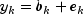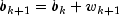k = 1, 2, 3, 4

where the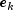s are identically and independently distributed normal with mean 0 and variance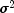, the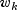s are identically and independently distributed normal with mean 0 and variance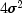, and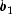is distributed normal with mean 4 and variance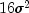. Two KalmanFilter objects are needed for each time point in order to compute the filtered estimate and the one-step-ahead estimate. The first object does not use the methods SetTransitionMatrix and setQ so that the prediction equations are skipped in the computations. The update equations are skipped in the computations in the second object.

This example also computes the one-step-ahead prediction errors. Harvey (1981, page 117) contains a misprint for the value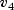that he gives as 1.197. The correct value of= 1.003 is computed by KalmanFilter.


import com.imsl.stat.*;
import java.text.MessageFormat;

public class KalmanFilterEx1 {

static private final MessageFormat mf
= new MessageFormat("{0}/{1}\t{2}\t{3}\t{4}\t{5}\t{6}\t{7}\t{8}");

public static void main(String args[]) {
int nobs = 4;
int rank = 0;
double logDeterminant = 0.0;
double ss = 0.0;
double[] b = {4};
double[][] covb = {{16}};
double[][] q = {{4}};
double[][] r = {{1}};
double[][] t = {{1}};
double[][] z = {{1}};
double[] ydata = {4.4, 4.0, 3.5, 4.6};

Object argFormat[] = {
"k", "j", "b", "cov(b)", "rank", "ss", "ln(det)", "v", "cov(v)"
};
System.out.println(mf.format(argFormat));

KalmanFilter kalman
= new KalmanFilter(b, covb, rank, ss, logDeterminant);

for (int i = 0; i < nobs; i++) {
double y[] = {ydata[i]};
kalman.update(y, z, r);
kalman.filter();
double v[] = kalman.getPredictionError();
double covv[][] = kalman.getCovV();
argFormat = new Integer(i);
argFormat = new Integer(i);
argFormat = new Double(kalman.getStateVector());
argFormat = new Double(kalman.getCovB());
argFormat = new Integer(kalman.getRank());
argFormat = new Double(kalman.getSumOfSquares());
argFormat = new Double(kalman.getLogDeterminant());
argFormat = new Double(v);
argFormat = new Double(covv);
System.out.println(mf.format(argFormat));
kalman.resetUpdate();

kalman.setTransitionMatrix(t);
kalman.setQ(q);
kalman.filter();
argFormat = new Integer(i + 1);
argFormat = new Integer(i);
argFormat = new Double(kalman.getStateVector());
argFormat = new Double(kalman.getCovB());
argFormat = new Integer(kalman.getRank());
argFormat = new Double(kalman.getSumOfSquares());
argFormat = new Double(kalman.getLogDeterminant());
argFormat = new Double(v);
argFormat = new Double(covv);
System.out.println(mf.format(argFormat));
kalman.resetTransitionMatrix();
kalman.resetQ();
}
}
}


#### Output

k/j	b	cov(b)	rank	ss	ln(det)	v	cov(v)
0/0	4.376	0.941	1	0.009	2.833	0.4	17
1/0	4.376	4.941	1	0.009	2.833	0.4	17
1/1	4.063	0.832	2	0.033	4.615	-0.376	5.941
2/1	4.063	4.832	2	0.033	4.615	-0.376	5.941
2/2	3.597	0.829	3	0.088	6.378	-0.563	5.832
3/2	3.597	4.829	3	0.088	6.378	-0.563	5.832
3/3	4.428	0.828	4	0.26	8.141	1.003	5.829
4/3	4.428	4.828	4	0.26	8.141	1.003	5.829